# Basics of Current

Current is classified into two types:

 � Direct Current (DC): DC is unidirectional flow of electric charge that means its direction remains constant. Examples of DC power source are battery and solar cell, etc. Direct Current or DC is suitable for using with DC appliances not to reverse polarity.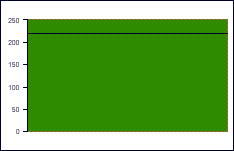� Alternative Current (AC): AC is an electric current which direction reverses cyclically. The usual waveform of AC power is sine wave with frequency 50 or 60 Hz. Examples of AC power sources are utility power supplies to residences and businesses, audio and radio signals, etc.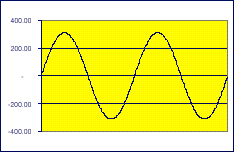Electrical power consumption
Electrical power consumed by DC electrical appliances can be determined by voltage that they work on and current that they consumed.

DC power consumption = Voltage (Volts) X Load Current (Amps) = Watts

Electrical power consumed by AC electrical appliances is more complicated than DC electrical appliances because the direction of current is changed periodically then the value of voltage and current should be measured in term of RMS (Root Mean Square) to eliminate changing of current direction.

AC electrical appliances or AC loads are classified into two types as the followings;

 � Linear load is electrical load consuming AC power both real power and apparent power with a power factor of 1. Example of linear load is incandescent lamp. � Non-linear load generates harmonic currents in addition to the original AC current and its power factor is less than 1. Examples of non-linear load are fluorescent lamp, electronic ballast of fluorescent lamp, PC and TV, etc.

Each type of AC load has different consuming current characteristic and the figures below show the current characteristic of linear load and non-linear load with same input voltage at same power rating of load.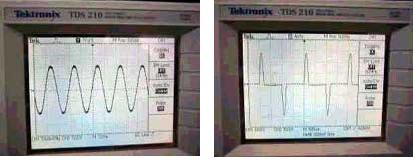Current characteristic ofincandescent lamp,which power factor = 1 Current characteristic ofa set of PC,which power factor = 0.52

What are Watt and VA?
Watt is unit of power. Its measures a rate of energy use or production and its symbol is W.
VA or volt-ampere is unit of electrical power consumed by non-linear load. It measures apparent power.

Example
A Computer is consumed power as a non-linear load then VA should be the measuring unit that indicates power consumed by PC.
A UPS is used to supply power to PC once the utility power is failed then a UPS should be declared its power in VA as well.

 � AC power (Watt) measuringTo measure real AC power of electrical appliances, the measuring equipment called "Power meter" is requested. This equipment will measure voltage and current at the same time and make calculation to get power in "Watt".The following figures show measuring of a PC with 17" monitor power consumption by using power meter.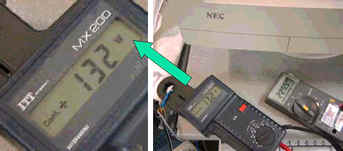� AC apparent power (VA) calculatingWe can measure the power of the same set of a PC with 17" monitor by measuring voltage (RMS) and current (RMS) after calculation power by multiply working voltage with consumed current.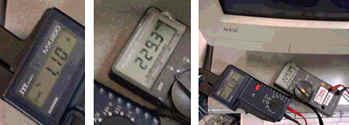AC apparent power = Voltage (RMS) X Load Current (RMS) = VA= 229.3 X 1.10 = 252.23 VA

 � The relation of AC power and AC apparent power AC power(Watts) = AC apparent power X Power factor= (VA) X Power factor

Power factor (pf) of an AC electrical power system is defined as ratio of real power to the apparent power and is a number between 0 and 1.

Power factor of linear load equals one (=1) and power factor of non-linear load is less than one (<1).

From a sample of a PC with 17" monitor, we can calculate power factor by

 Watts132pf = VA X pf= 252.23 X pf= 132 / 252.23 = 0.523